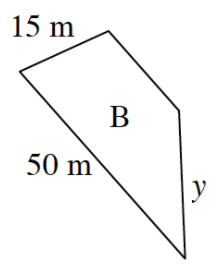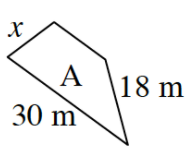### Home > CCAA > Chapter 5 Unit 3 > Lesson CC2: 5.1.2 > Problem5-22

5-22.

The trapezoids at right are similar shapes.

Refer to problem 4-94 for help with scale factors between two shapes.

1. What is the scale factor from shape A to shape B?

What is the ratio between the pair of sides $50$ m and $30$ m?

2. Find the lengths of the missing sides.

Use this ratio with each other pair of sides that only have one given length to solve for the variable.

$x=9$ m

If $x$ is $9$ m, what would $y$ be? Make sure you know how to get this answer.